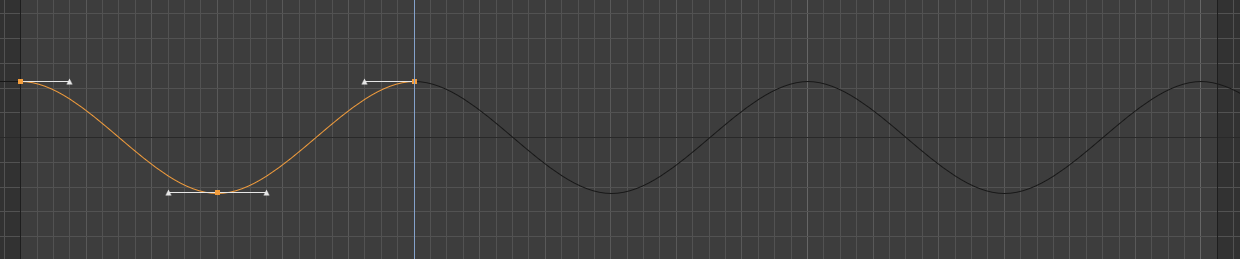# SOLVEDCCurve.GetValue() with Track Before & After Functions

Hi,
I'm using `CCurve.GetValue()`, but it only seems to work when there is an actual curve, not when there is a Track Before or After Function. Is there a way to get the value of the attribute with `CCurve.GetValue()` when using these functions?Thank you!

hi,

i create a simple example and it's working here. Just some precision issue (39.99999 instead of 40) but nothing too strange.
If you need to use the curve, you have to use the Remap function.

``````import c4d
from c4d import gui

# Main function
def main():
tracks = op.GetCTracks()
fps = doc.GetFps()
time  =  c4d.BaseTime(20, fps)
for track in tracks:
print track.GetValue(doc, time, fps)
curve = track.GetCurve(c4d.CCURVE_CURVE)
if curve:
newTime = track.Remap(time.Get())['ret_time']
newTime = c4d.BaseTime(newTime)
print curve.GetValue( newTime, fps)

# Execute main()
if __name__=='__main__':
main()
``````

Cheers,
Manuel

hi,

i create a simple example and it's working here. Just some precision issue (39.99999 instead of 40) but nothing too strange.
If you need to use the curve, you have to use the Remap function.

``````import c4d
from c4d import gui

# Main function
def main():
tracks = op.GetCTracks()
fps = doc.GetFps()
time  =  c4d.BaseTime(20, fps)
for track in tracks:
print track.GetValue(doc, time, fps)
curve = track.GetCurve(c4d.CCURVE_CURVE)
if curve:
newTime = track.Remap(time.Get())['ret_time']
newTime = c4d.BaseTime(newTime)
print curve.GetValue( newTime, fps)

# Execute main()
if __name__=='__main__':
main()
``````

Cheers,
Manuel

@m_magalhaes `Remap` did the trick! Thanks for the help, Manuel